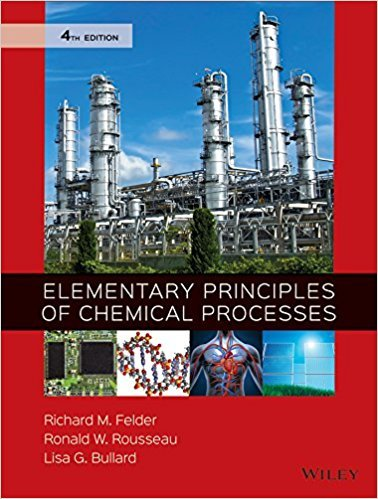×
×

# Solutions for Chapter 4.90: SUMMARY## Full solutions for Elementary Principles of Chemical Processes | 4th Edition

ISBN: 9780470616291Solutions for Chapter 4.90: SUMMARY

Solutions for Chapter 4.90
4 5 0 404 Reviews
24
4
##### ISBN: 9780470616291

Elementary Principles of Chemical Processes was written by and is associated to the ISBN: 9780470616291. Chapter 4.90: SUMMARY includes 1 full step-by-step solutions. Since 1 problems in chapter 4.90: SUMMARY have been answered, more than 41726 students have viewed full step-by-step solutions from this chapter. This expansive textbook survival guide covers the following chapters and their solutions. This textbook survival guide was created for the textbook: Elementary Principles of Chemical Processes, edition: 4.

Key Chemistry Terms and definitions covered in this textbook
• 1,2-elimination

An elimination reaction in which a proton from the beta (b) position is removed together with the leaving group, forming a double bond.

• allylic carbocation

A carbocation in which the positive charge is adjacent to a carbon-carbon double bond.

• base.

A substance that yields hydroxide ions (OH2) when dissolved in water. (2.7)

• biochemistry

The study of the chemistry of living systems. (Chapter 24: Introduction)

• chain branching

During polymerization, the growth of a branch connected to the main chain.

• chain reaction

A reaction (generally involving radicals) in which one chemical entity can ultimately cause a chemical transformation for thousands of molecules.

• coordination compound

A compound containing a metal ion bonded to a group of surrounding molecules or ions that act as ligands. (Section 23.2)

• donor atom

The atom of a ligand that bonds to the metal. (Section 23.2)

• E (Section 5.2C)

From the German, entgegen, opposite. Specifi es that groups of higher priority on the carbons of a double bond are on opposite sides

• element

A substance consisting of atoms of the same atomic number. Historically defined as a substance that cannot be separated into simpler substances by chemical means. (Sections 1.1 and 1.2)

• Hemiacetal

A molecule containing an !OH and an !OR or !OAr group bonded to the same carbon

• molar mass

The mass of one mole of a substance in grams; it is numerically equal to the formula weight in atomic mass units. (Section 3.4)

• octet rule

A rule stating that bonded atoms tend to possess or share a total of eight valence-shell electrons. (Section 8.1)

• pH titration curve

A graph of pH as a function of added titrant. (Section 17.3)

• phenoxide

The conjugate base of phenol or a substituted phenol.

• photodissociation

The breaking of a molecule into two or more neutral fragments as a result of absorption of light. (Section 18.2)

• Planck constant (h)

The constant that relates the energy and frequency of a photon, E = hn. Its value is 6.626 * 10-34 J@s. (Section 6.2)

• propagation

For radical reactions,the steps whose sum gives the net chemical reaction.

• standard atmospheric pressure

Defined as 760 torr or, in SI units, 101.325 kPa. (Section 10.2)

• Tesla (T)

The SI unit for magnetic fi eld strength.

×